Скачать презентацию Lower Bounds for Local Search by Quantum Arguments

bf11dec9104c7809192f8444d6e2c811.ppt

• Количество слайдов: 25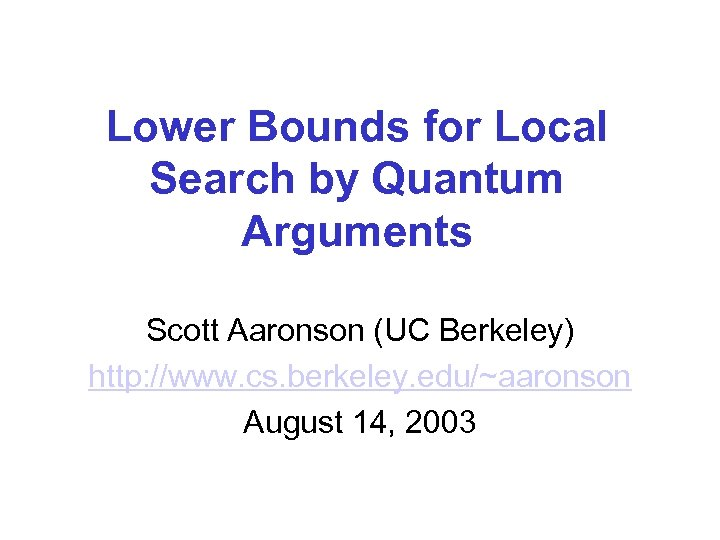Lower Bounds for Local Search by Quantum Arguments Scott Aaronson (UC Berkeley) http: //www. cs. berkeley. edu/~aaronson August 14, 2003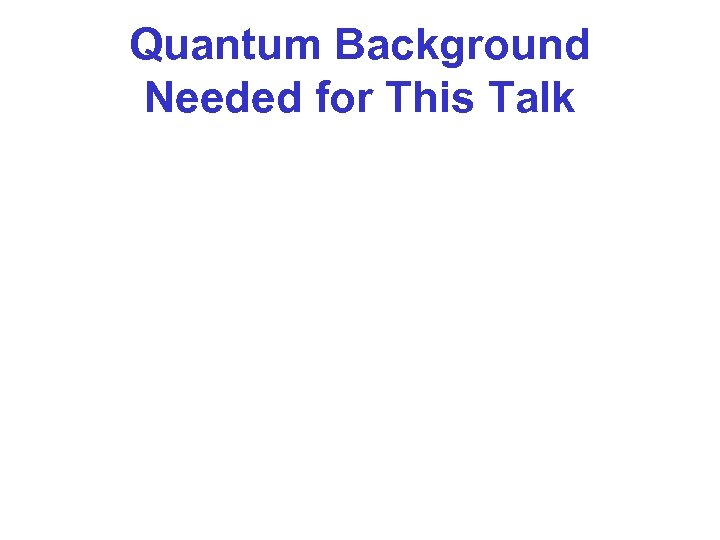Quantum Background Needed for This Talk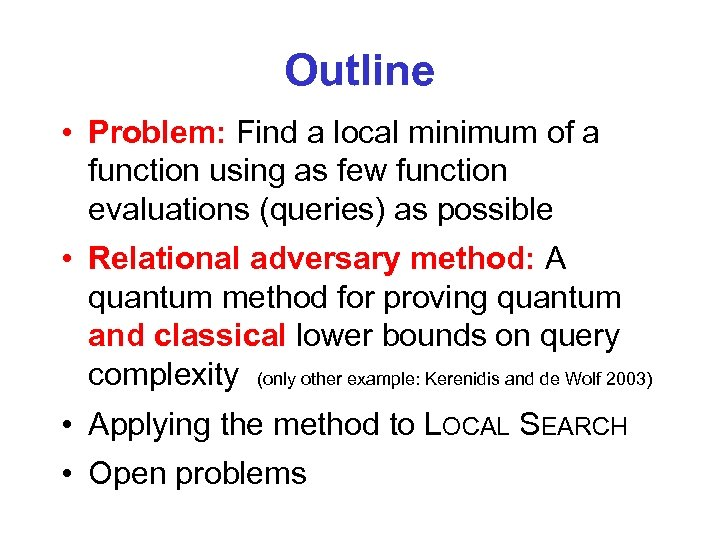Outline • Problem: Find a local minimum of a function using as few function evaluations (queries) as possible • Relational adversary method: A quantum method for proving quantum and classical lower bounds on query complexity (only other example: Kerenidis and de Wolf 2003) • Applying the method to LOCAL SEARCH • Open problems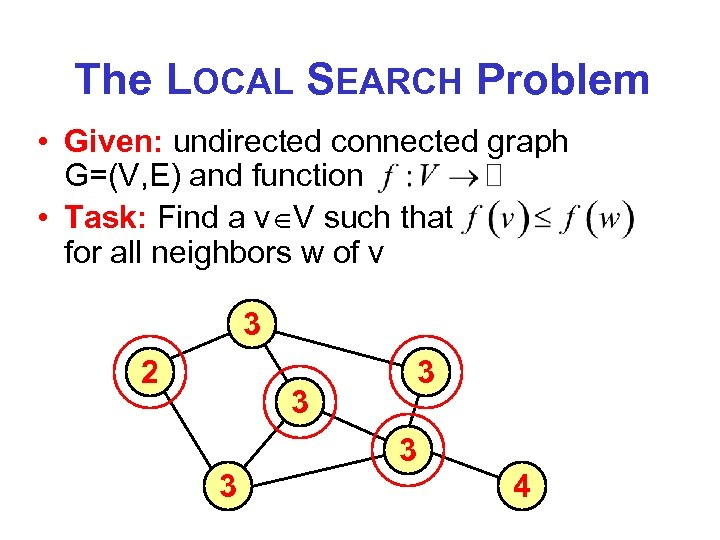The LOCAL SEARCH Problem • Given: undirected connected graph G=(V, E) and function • Task: Find a v V such that for all neighbors w of v 3 2 3 3 4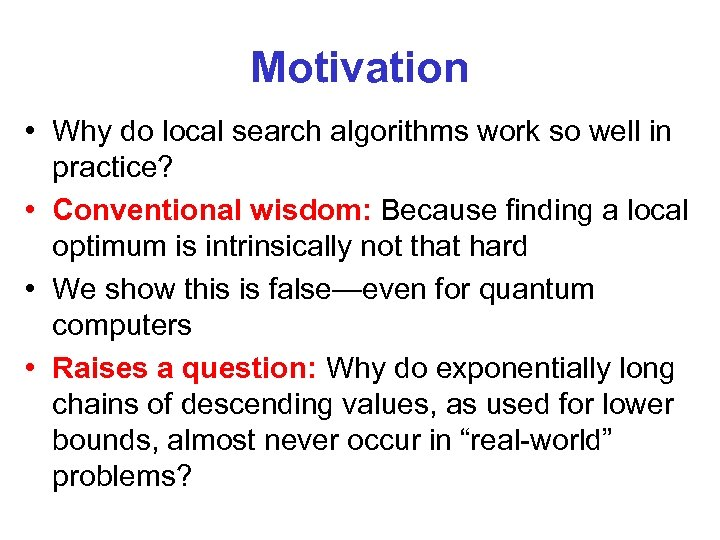Motivation • Why do local search algorithms work so well in practice? • Conventional wisdom: Because finding a local optimum is intrinsically not that hard • We show this is false—even for quantum computers • Raises a question: Why do exponentially long chains of descending values, as used for lower bounds, almost never occur in “real-world” problems?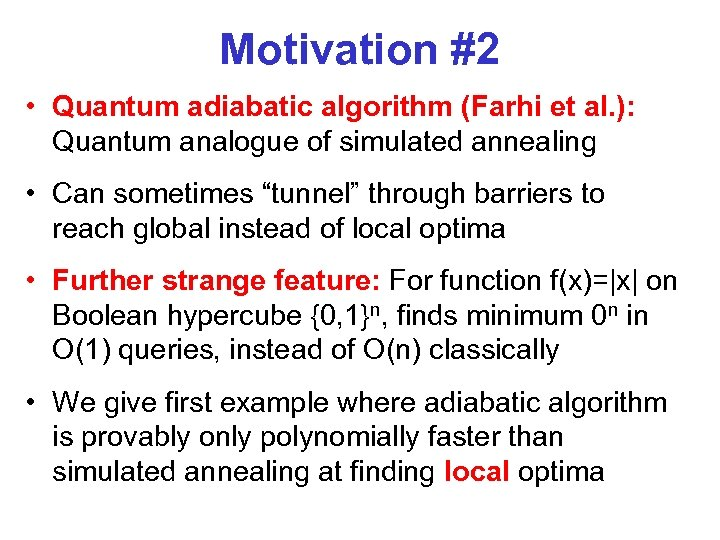Motivation #2 • Quantum adiabatic algorithm (Farhi et al. ): Quantum analogue of simulated annealing • Can sometimes “tunnel” through barriers to reach global instead of local optima • Further strange feature: For function f(x)=|x| on Boolean hypercube {0, 1}n, finds minimum 0 n in O(1) queries, instead of O(n) classically • We give first example where adiabatic algorithm is provably only polynomially faster than simulated annealing at finding local optima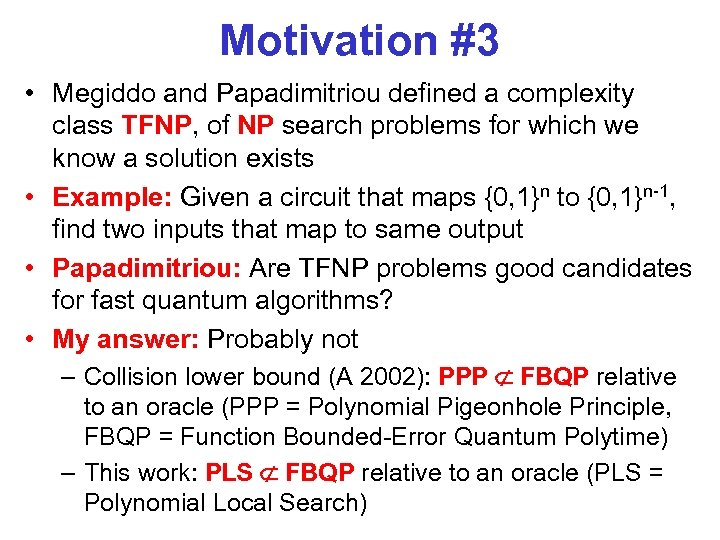Motivation #3 • Megiddo and Papadimitriou defined a complexity class TFNP, of NP search problems for which we know a solution exists • Example: Given a circuit that maps {0, 1}n to {0, 1}n-1, find two inputs that map to same output • Papadimitriou: Are TFNP problems good candidates for fast quantum algorithms? • My answer: Probably not – Collision lower bound (A 2002): PPP FBQP relative to an oracle (PPP = Polynomial Pigeonhole Principle, FBQP = Function Bounded-Error Quantum Polytime) – This work: PLS FBQP relative to an oracle (PLS = Polynomial Local Search)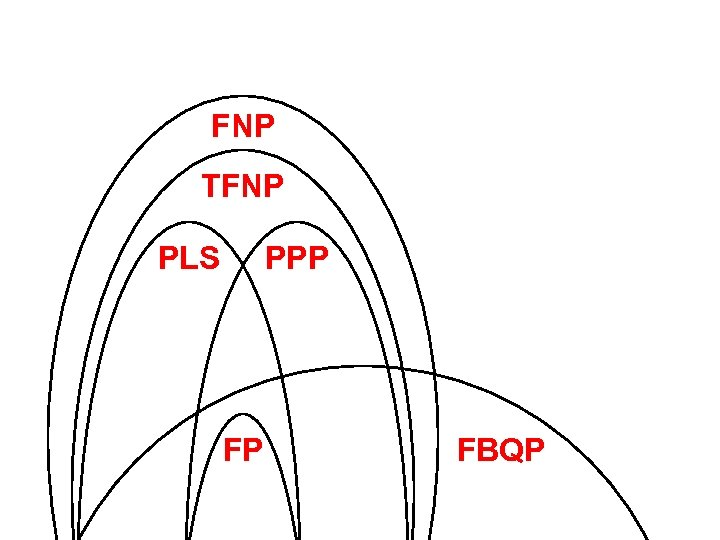FNP TFNP PLS PPP FP FBQP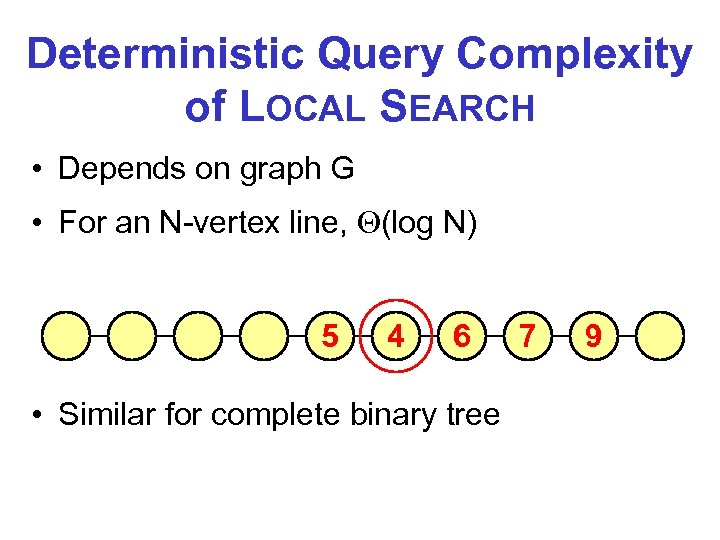Deterministic Query Complexity of LOCAL SEARCH • Depends on graph G • For an N-vertex line, (log N) 5 4 6 • Similar for complete binary tree 7 9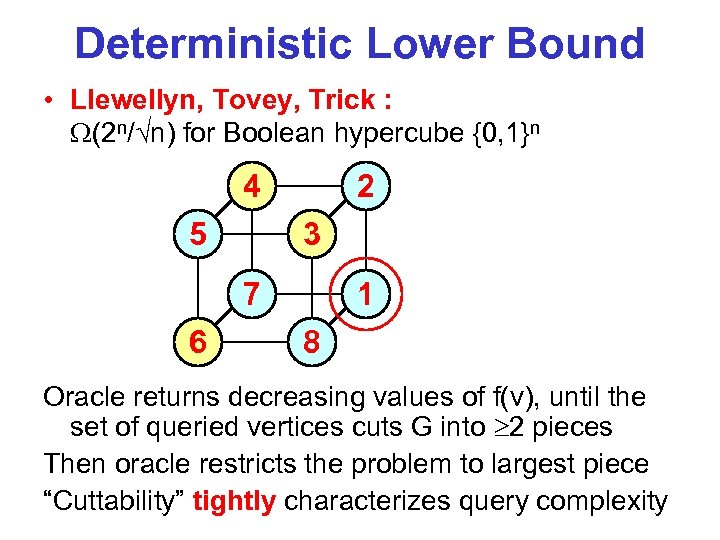Deterministic Lower Bound • Llewellyn, Tovey, Trick : (2 n/ n) for Boolean hypercube {0, 1}n 4 5 2 3 7 6 1 8 Oracle returns decreasing values of f(v), until the set of queried vertices cuts G into 2 pieces Then oracle restricts the problem to largest piece “Cuttability” tightly characterizes query complexity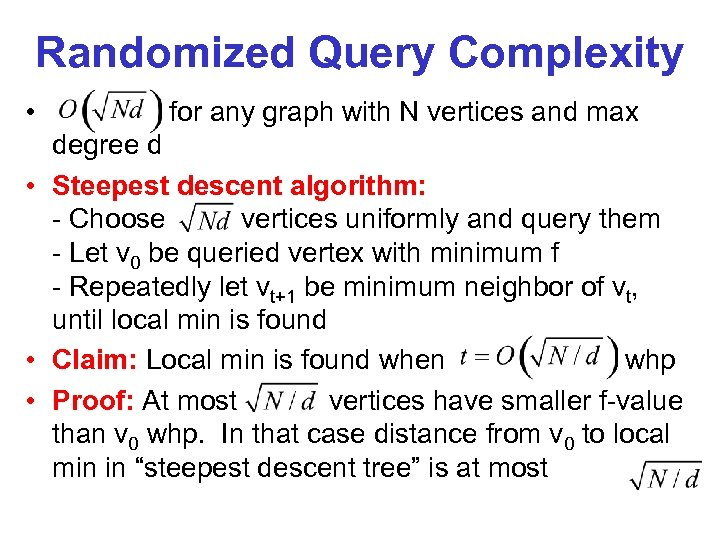Randomized Query Complexity • for any graph with N vertices and max degree d • Steepest descent algorithm: - Choose vertices uniformly and query them - Let v 0 be queried vertex with minimum f - Repeatedly let vt+1 be minimum neighbor of vt, until local min is found • Claim: Local min is found when whp • Proof: At most vertices have smaller f-value than v 0 whp. In that case distance from v 0 to local min in “steepest descent tree” is at most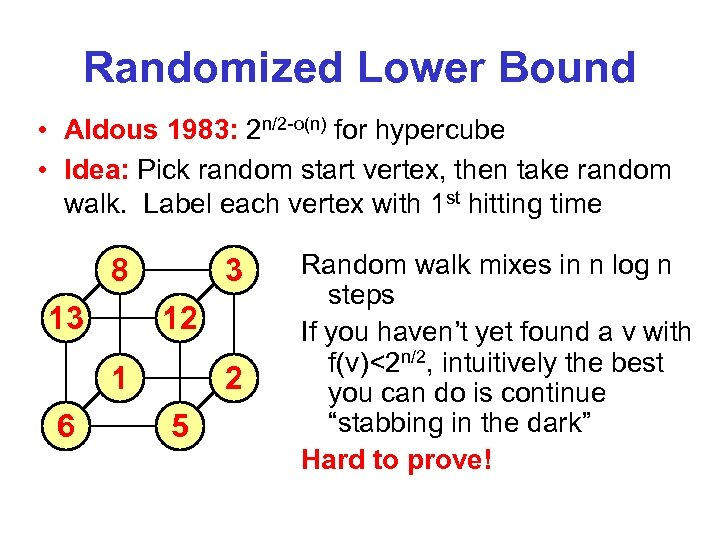Randomized Lower Bound • Aldous 1983: 2 n/2 -o(n) for hypercube • Idea: Pick random start vertex, then take random walk. Label each vertex with 1 st hitting time 8 13 3 12 1 6 2 5 Random walk mixes in n log n steps If you haven’t yet found a v with f(v)<2 n/2, intuitively the best you can do is continue “stabbing in the dark” Hard to prove!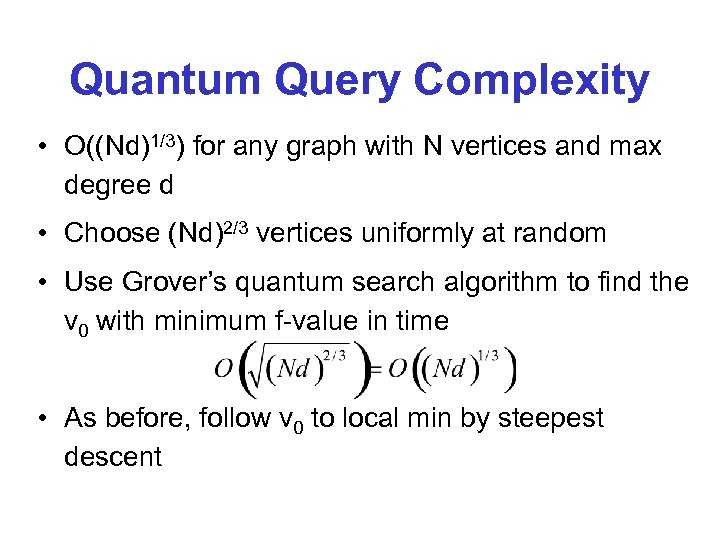Quantum Query Complexity • O((Nd)1/3) for any graph with N vertices and max degree d • Choose (Nd)2/3 vertices uniformly at random • Use Grover’s quantum search algorithm to find the v 0 with minimum f-value in time • As before, follow v 0 to local min by steepest descent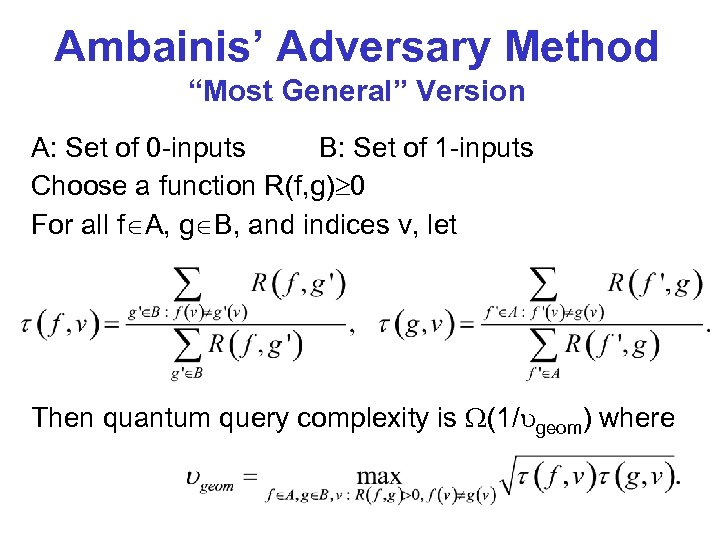Ambainis’ Adversary Method “Most General” Version A: Set of 0 -inputs B: Set of 1 -inputs Choose a function R(f, g) 0 For all f A, g B, and indices v, let Then quantum query complexity is (1/ geom) where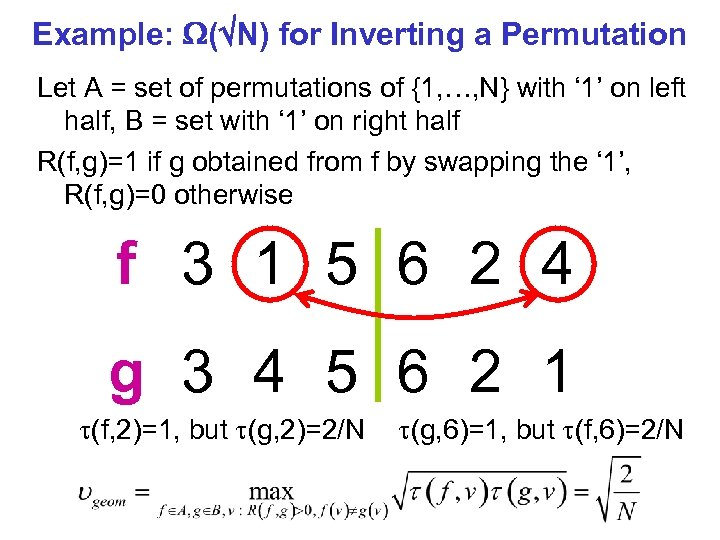Example: ( N) for Inverting a Permutation Let A = set of permutations of {1, …, N} with ‘ 1’ on left half, B = set with ‘ 1’ on right half R(f, g)=1 if g obtained from f by swapping the ‘ 1’, R(f, g)=0 otherwise f 3 1 5 6 2 4 g 3 4 5 6 2 1 (f, 2)=1, but (g, 2)=2/N (g, 6)=1, but (f, 6)=2/N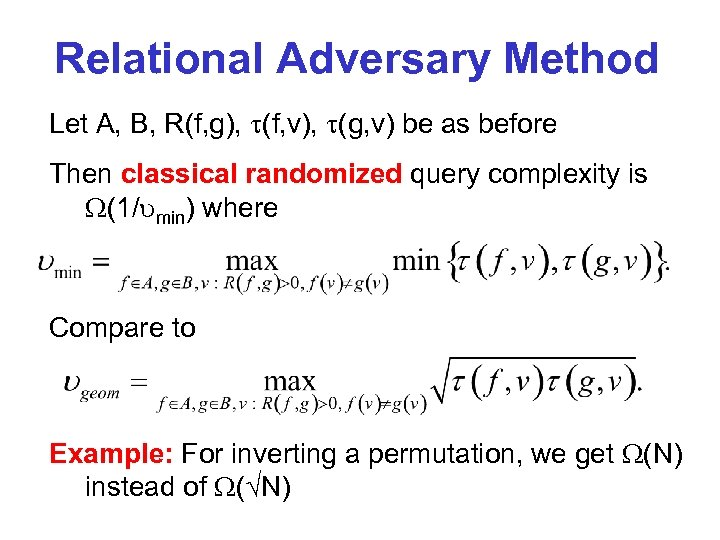Relational Adversary Method Let A, B, R(f, g), (f, v), (g, v) be as before Then classical randomized query complexity is (1/ min) where Compare to Example: For inverting a permutation, we get (N) instead of ( N)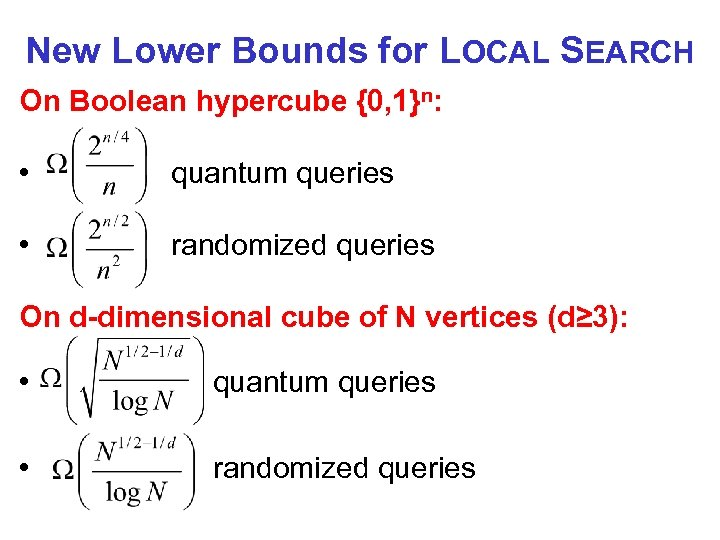New Lower Bounds for LOCAL SEARCH On Boolean hypercube {0, 1}n: • quantum queries • randomized queries On d-dimensional cube of N vertices (d≥ 3): • quantum queries • randomized queries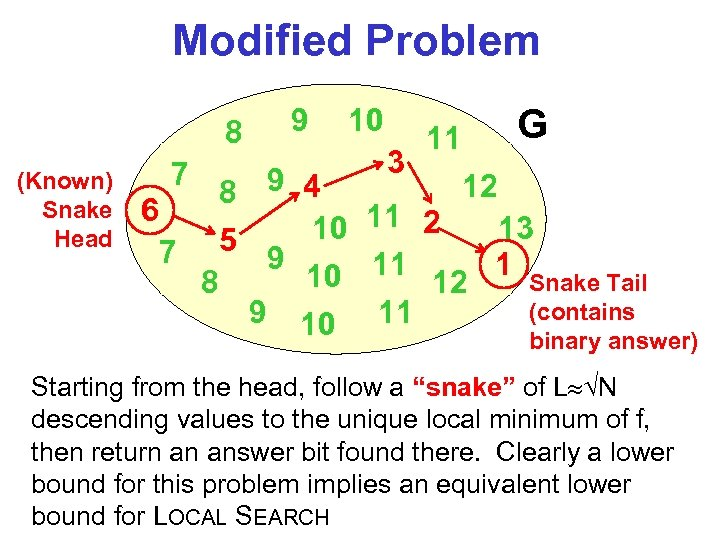Modified Problem 8 (Known) Snake Head 7 9 10 3 11 G 12 8 9 4 6 10 11 2 13 5 7 9 10 11 12 1 Snake Tail 8 (contains 9 10 11 binary answer) Starting from the head, follow a “snake” of L N descending values to the unique local minimum of f, then return an answer bit found there. Clearly a lower bound for this problem implies an equivalent lower bound for LOCAL SEARCH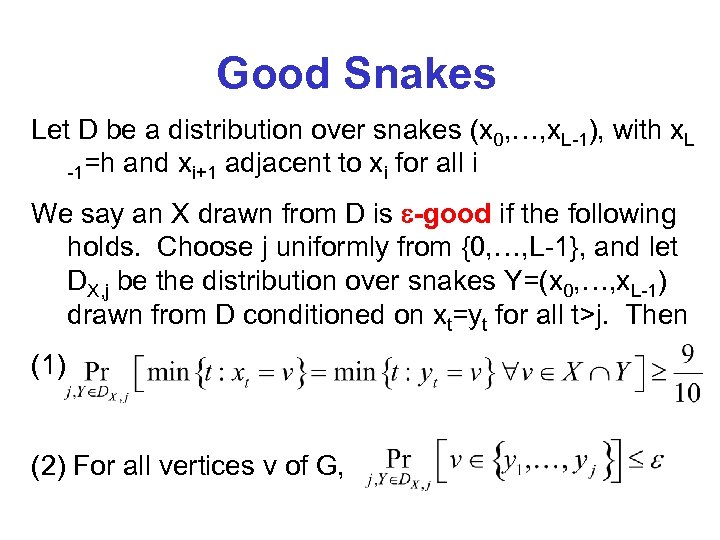Good Snakes Let D be a distribution over snakes (x 0, …, x. L-1), with x. L -1=h and xi+1 adjacent to xi for all i We say an X drawn from D is -good if the following holds. Choose j uniformly from {0, …, L-1}, and let DX, j be the distribution over snakes Y=(x 0, …, x. L-1) drawn from D conditioned on xt=yt for all t>j. Then (1) (2) For all vertices v of G,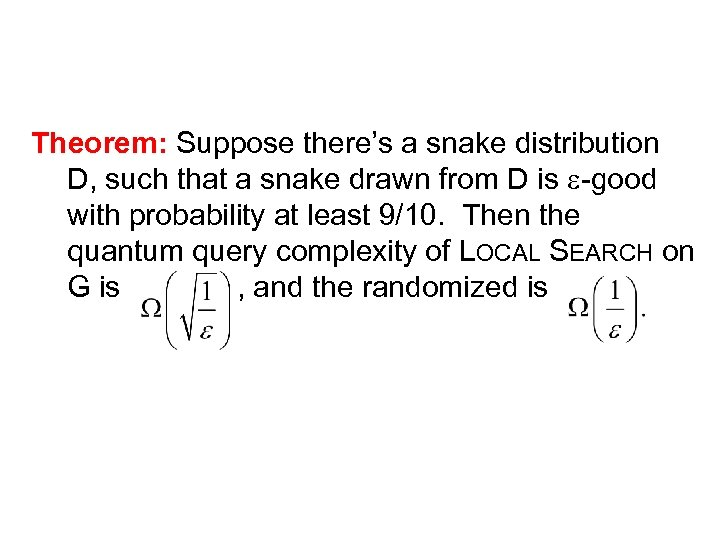Theorem: Suppose there’s a snake distribution D, such that a snake drawn from D is -good with probability at least 9/10. Then the quantum query complexity of LOCAL SEARCH on G is , and the randomized is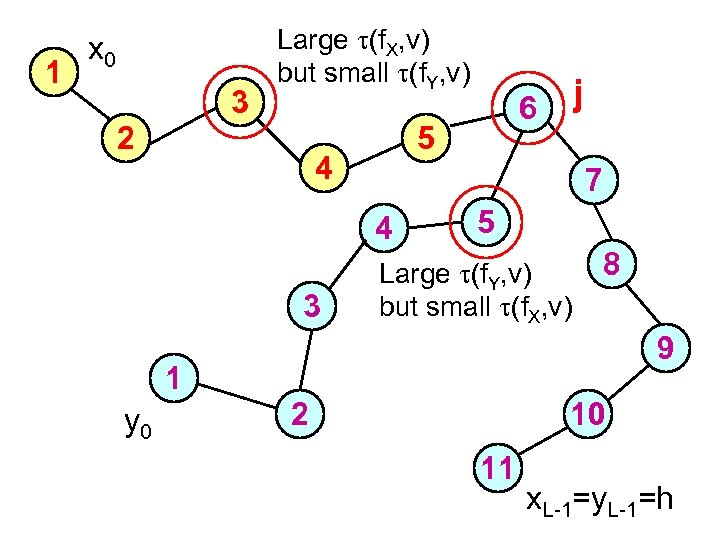1 x 0 3 Large (f. X, v) but small (f. Y, v) 2 5 4 7 4 3 j 6 5 Large (f. Y, v) but small (f. X, v) 8 9 1 y 0 2 10 11 x. L-1=y. L-1=h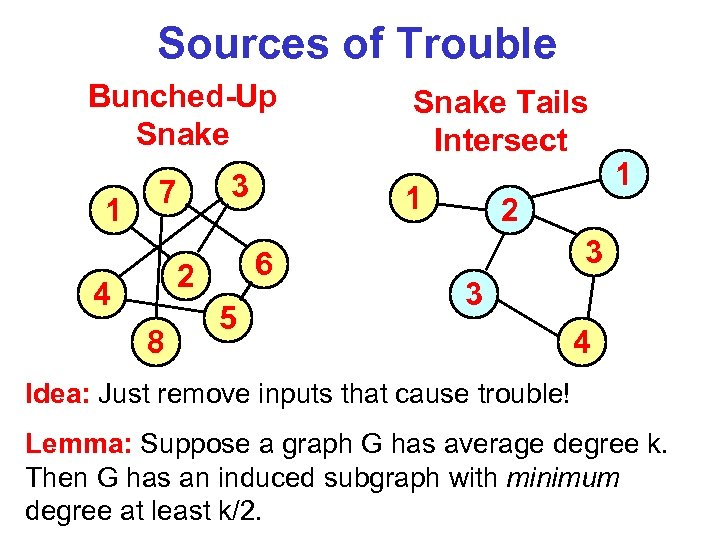Sources of Trouble Bunched-Up Snake 1 3 7 4 8 1 6 2 5 Snake Tails Intersect 1 2 3 3 4 Idea: Just remove inputs that cause trouble! Lemma: Suppose a graph G has average degree k. Then G has an induced subgraph with minimum degree at least k/2.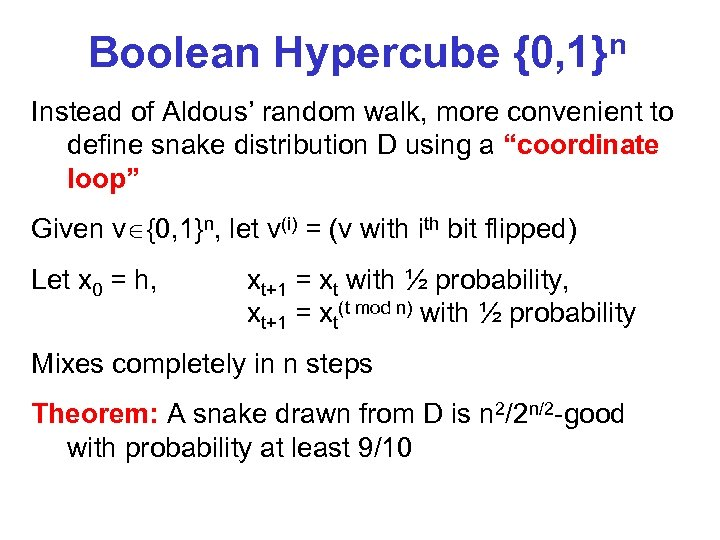Boolean Hypercube {0, 1}n Instead of Aldous’ random walk, more convenient to define snake distribution D using a “coordinate loop” Given v {0, 1}n, let v(i) = (v with bit flipped) Let x 0 = h, xt+1 = xt with ½ probability, xt+1 = xt(t mod n) with ½ probability Mixes completely in n steps Theorem: A snake drawn from D is n 2/2 n/2 -good with probability at least 9/10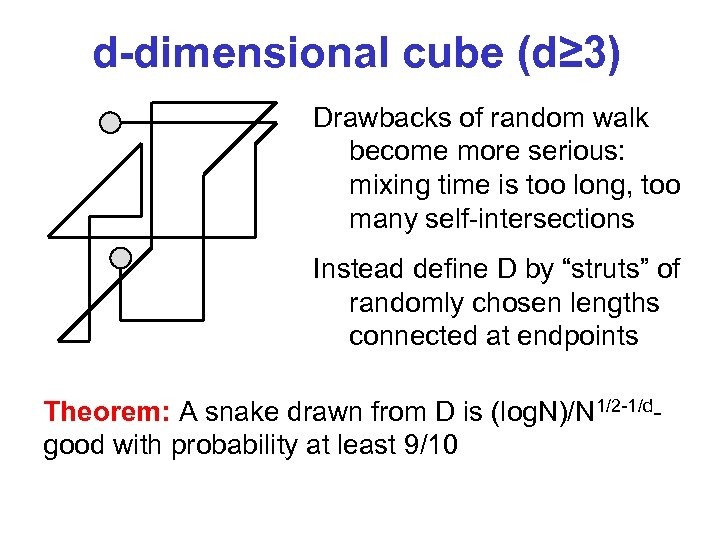d-dimensional cube (d≥ 3) Drawbacks of random walk become more serious: mixing time is too long, too many self-intersections Instead define D by “struts” of randomly chosen lengths connected at endpoints Theorem: A snake drawn from D is (log. N)/N 1/2 -1/dgood with probability at least 9/10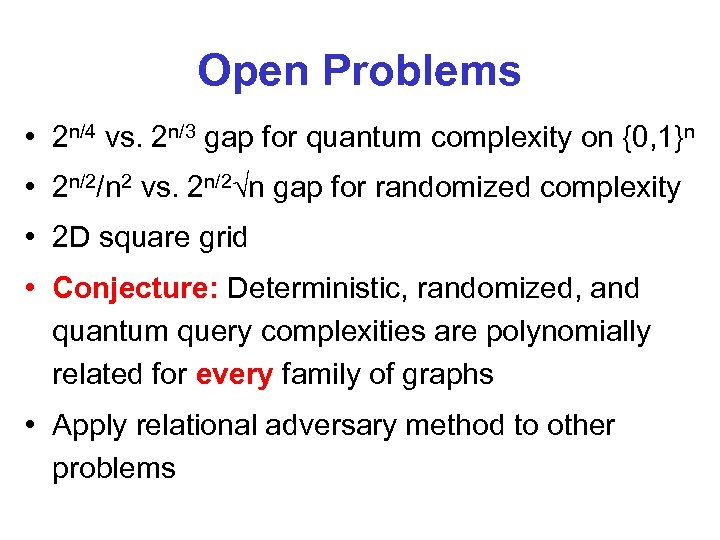Open Problems • 2 n/4 vs. 2 n/3 gap for quantum complexity on {0, 1}n • 2 n/2/n 2 vs. 2 n/2 n gap for randomized complexity • 2 D square grid • Conjecture: Deterministic, randomized, and quantum query complexities are polynomially related for every family of graphs • Apply relational adversary method to other problems